$\newcommand{\NN}{\mathbb{N}} \newcommand{\CC}{\mathbb{C}} \newcommand{\GG}{\mathbb{G}} \newcommand{\LL}{\mathbb{L}} \newcommand{\PP}{\mathbb{P}} \newcommand{\QQ}{\mathbb{Q}} \newcommand{\RR}{\mathbb{R}} \newcommand{\VV}{\mathbb{V}} \newcommand{\ZZ}{\mathbb{Z}} \newcommand{\FF}{\mathbb{F}} \newcommand{\KK}{\mathbb{K}} \newcommand{\UU}{\mathbb{U}} \newcommand{\EE}{\mathbb{E}} \newcommand{\Aa}{\mathcal{A}} \newcommand{\Bb}{\mathcal{B}} \newcommand{\Cc}{\mathcal{C}} \newcommand{\Dd}{\mathcal{D}} \newcommand{\Ee}{\mathcal{E}} \newcommand{\Ff}{\mathcal{F}} \newcommand{\Gg}{\mathcal{G}} \newcommand{\Hh}{\mathcal{H}} \newcommand{\Ii}{\mathcal{I}} \newcommand{\Jj}{\mathcal{J}} \newcommand{\Kk}{\mathcal{K}} \newcommand{\Ll}{\mathcal{L}} \newcommand{\Mm}{\mathcal{M}} \newcommand{\Nn}{\mathcal{N}} \newcommand{\Oo}{\mathcal{O}} \newcommand{\Pp}{\mathcal{P}} \newcommand{\Qq}{\mathcal{Q}} \newcommand{\Rr}{\mathcal{R}} \newcommand{\Ss}{\mathcal{S}} \newcommand{\Tt}{\mathcal{T}} \newcommand{\Uu}{\mathcal{U}} \newcommand{\Vv}{\mathcal{V}} \newcommand{\Ww}{\mathcal{W}} \newcommand{\Xx}{\mathcal{X}} \newcommand{\Yy}{\mathcal{Y}} \newcommand{\Zz}{\mathcal{Z}} \newcommand{\al}{\alpha} \newcommand{\la}{\lambda} \newcommand{\ga}{\gamma} \newcommand{\Ga}{\Gamma} \newcommand{\La}{\Lambda} \newcommand{\Si}{\Sigma} \newcommand{\si}{\sigma} \newcommand{\be}{\beta} \newcommand{\de}{\delta} \newcommand{\De}{\Delta} \renewcommand{\phi}{\varphi} \renewcommand{\th}{\theta} \newcommand{\om}{\omega} \newcommand{\Om}{\Omega} \renewcommand{\epsilon}{\varepsilon} \newcommand{\Calpha}{\mathrm{C}^\al} \newcommand{\Cbeta}{\mathrm{C}^\be} \newcommand{\Cal}{\text{C}^\al} \newcommand{\Cdeux}{\text{C}^{2}} \newcommand{\Cun}{\text{C}^{1}} \newcommand{\Calt}{\text{C}^{#1}} \newcommand{\lun}{\ell^1} \newcommand{\ldeux}{\ell^2} \newcommand{\linf}{\ell^\infty} \newcommand{\ldeuxj}{{\ldeux_j}} \newcommand{\Lun}{\text{\upshape L}^1} \newcommand{\Ldeux}{\text{\upshape L}^2} \newcommand{\Lp}{\text{\upshape L}^p} \newcommand{\Lq}{\text{\upshape L}^q} \newcommand{\Linf}{\text{\upshape L}^\infty} \newcommand{\lzero}{\ell^0} \newcommand{\lp}{\ell^p} \renewcommand{\d}{\ins{d}} \newcommand{\Grad}{\text{Grad}} \newcommand{\grad}{\text{grad}} \renewcommand{\div}{\text{div}} \newcommand{\diag}{\text{diag}} \newcommand{\pd}{ \frac{ \partial #1}{\partial #2} } \newcommand{\pdd}{ \frac{ \partial^2 #1}{\partial #2^2} } \newcommand{\dotp}{\langle #1,\,#2\rangle} \newcommand{\norm}{|\!| #1 |\!|} \newcommand{\normi}{\norm{#1}_{\infty}} \newcommand{\normu}{\norm{#1}_{1}} \newcommand{\normz}{\norm{#1}_{0}} \newcommand{\abs}{\vert #1 \vert} \newcommand{\argmin}{\text{argmin}} \newcommand{\argmax}{\text{argmax}} \newcommand{\uargmin}{\underset{#1}{\argmin}\;} \newcommand{\uargmax}{\underset{#1}{\argmax}\;} \newcommand{\umin}{\underset{#1}{\min}\;} \newcommand{\umax}{\underset{#1}{\max}\;} \newcommand{\pa}{\left( #1 \right)} \newcommand{\choice}{ \left\{ \begin{array}{l} #1 \end{array} \right. } \newcommand{\enscond}{ \left\{ #1 \;:\; #2 \right\} } \newcommand{\qandq}{ \quad \text{and} \quad } \newcommand{\qqandqq}{ \qquad \text{and} \qquad } \newcommand{\qifq}{ \quad \text{if} \quad } \newcommand{\qqifqq}{ \qquad \text{if} \qquad } \newcommand{\qwhereq}{ \quad \text{where} \quad } \newcommand{\qqwhereqq}{ \qquad \text{where} \qquad } \newcommand{\qwithq}{ \quad \text{with} \quad } \newcommand{\qqwithqq}{ \qquad \text{with} \qquad } \newcommand{\qforq}{ \quad \text{for} \quad } \newcommand{\qqforqq}{ \qquad \text{for} \qquad } \newcommand{\qqsinceqq}{ \qquad \text{since} \qquad } \newcommand{\qsinceq}{ \quad \text{since} \quad } \newcommand{\qarrq}{\quad\Longrightarrow\quad} \newcommand{\qqarrqq}{\quad\Longrightarrow\quad} \newcommand{\qiffq}{\quad\Longleftrightarrow\quad} \newcommand{\qqiffqq}{\qquad\Longleftrightarrow\qquad} \newcommand{\qsubjq}{ \quad \text{subject to} \quad } \newcommand{\qqsubjqq}{ \qquad \text{subject to} \qquad }$

This numerical tour explores the reconstruction from 3D tomographic measurement with TV regularization.

## Installing toolboxes and setting up the path.

You need to download the following files: signal toolbox and general toolbox.

You need to unzip these toolboxes in your working directory, so that you have toolbox_signal and toolbox_general in your directory.

For Scilab user: you must replace the Matlab comment '%' by its Scilab counterpart '//'.

Recommandation: You should create a text file named for instance numericaltour.sce (in Scilab) or numericaltour.m (in Matlab) to write all the Scilab/Matlab command you want to execute. Then, simply run exec('numericaltour.sce'); (in Scilab) or numericaltour; (in Matlab) to run the commands.

Execute this line only if you are using Matlab.

getd = @(p)path(p,path); % scilab users must *not* execute this


Then you can add the toolboxes to the path.

getd('toolbox_signal/');
getd('toolbox_general/');


## 3D Volumetric Datasets

name = 'vessels';
options.nbdims = 3;
M = rescale(M);
% size of the image (here it is a cube).
n = size(M,1);


Reduce dimensionality

M = M(1:2:n,1:2:n,1:2:n);
n = n/2;


We can display some horizontal slices.

slices = round(linspace(10,n-10,4));
clf;
for i=1:length(slices)
s = slices(i);
imageplot( M(:,:,s), strcat(['Z=' num2str(s)]), 2,2,i );
end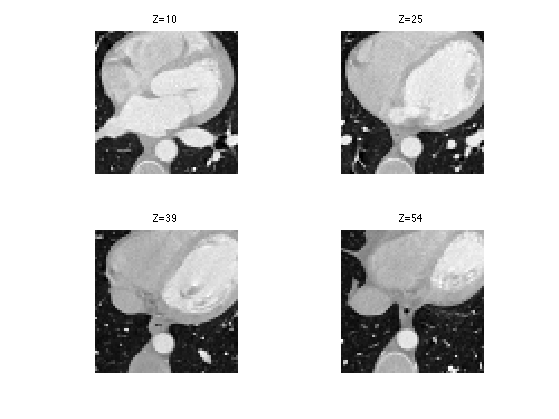We can display an isosurface of the dataset (here we sub-sample to speed up the computation).

sel = 1:2:n;
clf;
isosurface( M(sel,sel,sel), .5);
axis('off');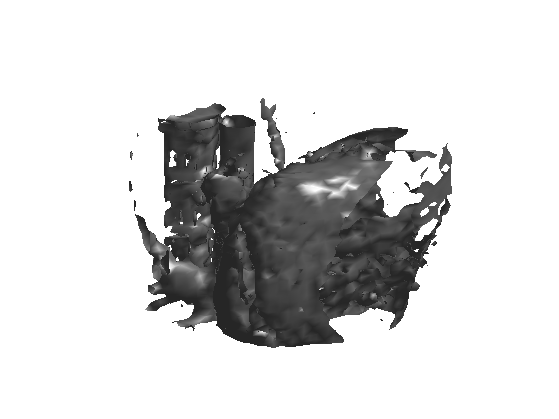## 3D Tomograpic Measurements

Tomographic measurements corresponds to the computation of orthogonal projections (integration along liners) of the 3D datasets on set of 2D planes. Thanks to the Fourier-slice theorem, this is equivalent to performing a sub-sampling of the 3D Fourier transform along planes (orthogonal to the projection directions).

Number of projections

P = 12;


Either uniform sampling for P==12, or randomized projection directions, on the sphere.

if P==12
tau = 0.8506508084;
one = 0.5257311121;
S = [  tau,  one,    0;
-tau,  one,    0
-tau, -one,    0;
tau, -one,    0;
one,   0 ,  tau;
one,   0 , -tau;
-one,   0 , -tau;
-one,   0 ,  tau;
0 ,  tau,  one;
0 , -tau,  one;
0 , -tau, -one;
0 ,  tau, -one ]';
else
S = randn(3,P);
S = S ./ repmat( sqrt( sum(S.^2,1) ), [3 1]);
end


Display the directions on the sphere.

clf;
plot3(S(1,:), S(2,:), S(3,:), '.');
axis equal;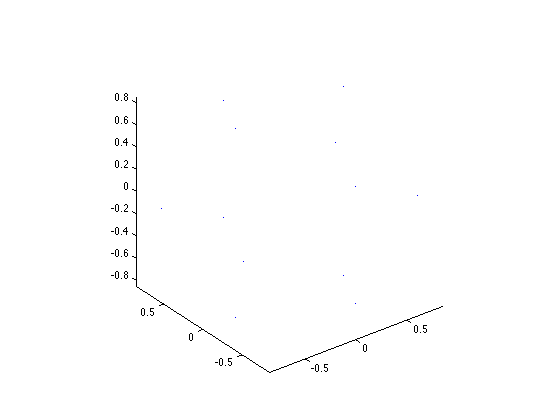x = [0:n/2-1, -n/2:-1];
[X,Y,Z] = ndgrid(x,x,x);
epsilon = .5;
for i=1:P
q = S(:,i);
d = q(1)*X + q(2)*Y + q(3)*Z;
end


Tomographic measurement can thus be intepreted as a selection of a few Fourier frequencies.

F = fftn(M);


Number of measures.

Q = length(y);
disp(strcat(['Number of measurements Q=' num2str(Q) '.']));
disp(strcat(['Sub-sampling Q/N=' num2str(length(y)/n^3,2) '.']));

Number of measurements Q=27906.
Sub-sampling Q/N=0.11.


The transposed operator corresponds to the pseudo inverse reconstruction (because the measurement operator is in fact an orthogonal projection). It is similar to the filtered back-projection (excepted that the Fourier sub-sampling is now on a discrete grid, which is not really faithful to the geometry of tomographic acquisition).

F1 = zeros(n,n,n);
M1 = real( ifftn(F1) );


Display.

clf;
for i=1:length(slices)
s = slices(i);
imageplot( clamp(M1(:,:,s)), strcat(['Z=' num2str(s)]), 2,2,i );
end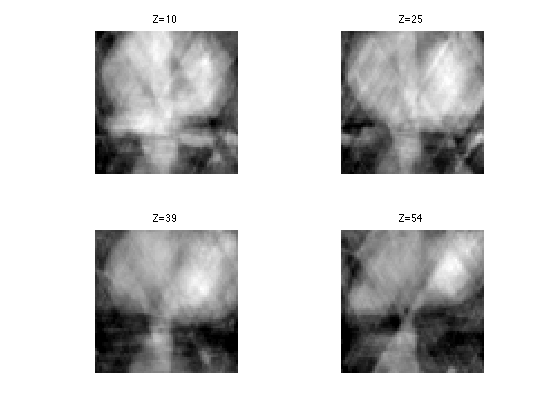SNR of the pseudo inverse reconstruction.

disp(['Pseudo-inverse, SNR=' num2str(snr(M,M1),4) 'dB.']);

Pseudo-inverse, SNR=11.89dB.


## 3D Total Variation

Since medical images often have a cartoon morphology, it makes sense to perform the reconstruction while minimizing the TV norm of the image.

To be able to use a gradient descent for the reconstruction, we use a smoothed TV-norm, using a smoothing parameter epsilon. The smaller, the closer to TV, but the slower the method is.

epsilon = 1e-2;


The gradient of the volum is a 3D vector field, and hence a (n,n,n,3) matrix.

G = grad(M);


Display the gradient as a color image.

clf;
for i=1:length(slices)
s = slices(i);
imageplot( squeeze(G(:,:,s,:)), strcat(['Z=' num2str(s)]), 2,2,i );
end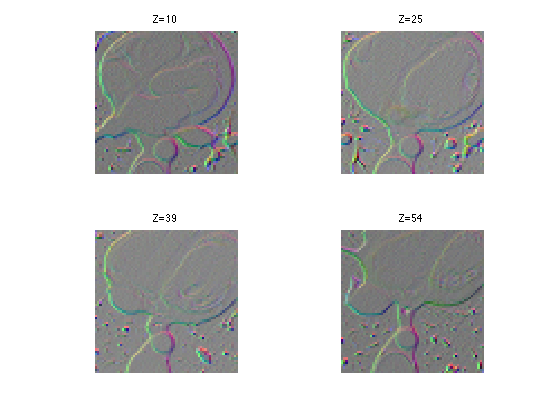Compute the smoothed norm of the gradient. This corresponds to a edge detector.

d = sqrt(sum(G.^2,4)+epsilon^2);


Display.

clf;
for i=1:length(slices)
s = slices(i);
imageplot( squeeze(d(:,:,s,:)), strcat(['Z=' num2str(s)]), 2,2,i );
end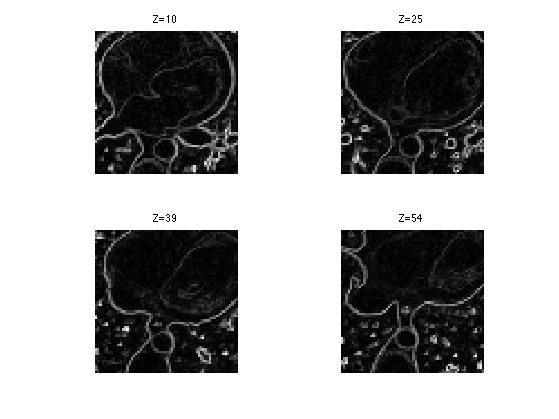Compute the regularized TV norm.

tv = sum(d(:));
disp(['TV norm=' num2str(tv) '.']);

TV norm=20070.761.


## TV Reconstruction from Partial 3D Tomoraphic Measurements

We perform the reconstruction using a projected gradient descent.

Gradient descent step. Should be proportional to epsilon.

tau = epsilon*.2;


Initialize the solution using the pseudo inverse.

Mtv = M1;


The gradient of the smoothed total variation is minus the divergence of the normalized gradient of the image.

G = grad(Mtv);
d = sqrt(sum(G.^2,4)+epsilon^2);
dG = -div( G ./ repmat(d, [1 1 1 3]) );


Display it.

clf;
for i=1:length(slices)
s = slices(i);
imageplot( dG(:,:,s), strcat(['Z=' num2str(s)]), 2,2,i );
end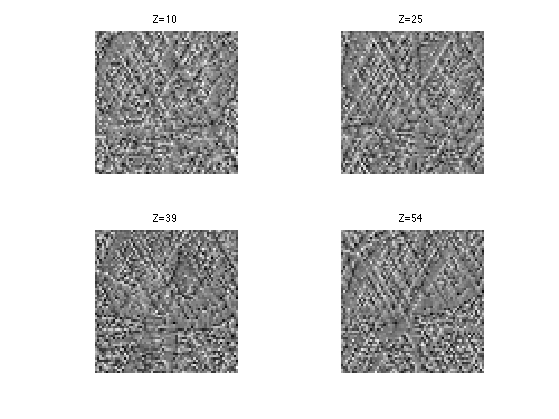Mtv = Mtv - tau*dG;


Perform the projection step to impose the known values.

F = fftn(Mtv);
Mtv = real(ifftn(F));


Exercice 1: (check the solution) Perform the projected TV gradient desent, and monitor the epsilon-TV norm during the iterations.

exo1;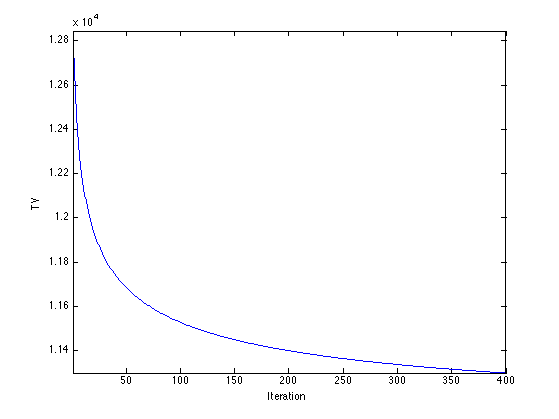Display.

clf;
for i=1:length(slices)
s = slices(i);
imageplot( clamp(Mtv(:,:,s)), strcat(['Z=' num2str(s)]), 2,2,i );
end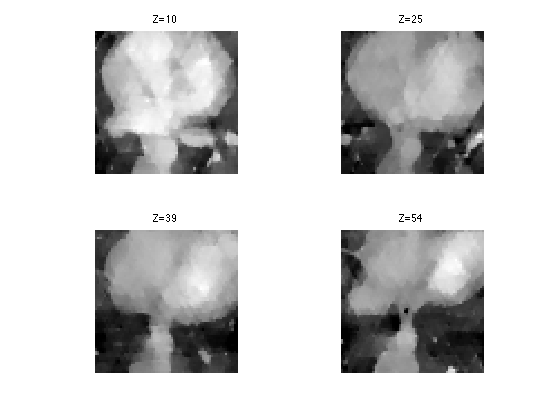SNR of the resulting reconstruction.

disp(['Total variation, SNR=' num2str(snr(M,Mtv),4) 'dB.']);

Total variation, SNR=13.94dB.